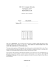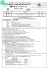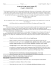Automata Theory Sample Midterm Exam

Transcription

Automata Theory Sample Midterm Exam
Automata Theory
Sample Midterm Exam
1. (20 pts) Mark by T(=True) or F(=False) each of the following statements. You don’t need
to prove it.
(1) There are languages for which L∗ = (L)∗
R
(2) (L1 L2 )R = LR
2 L1 for all languages L1 and L2 .
(3) (LR )∗ = (L∗ )R for all languages L.
(4) Let L = {awa : a ∈ {a, b}∗ }. Then, L∗ is regular.
2. (5 pts) Show that S1 = S2 ⇔ (S1 ∩ S2 ) ∪ (S1 ∩ S2 ) = ∅.
3. (25 pts) Please give the answer to each of the following questions. You do not need to show
how to derive the answers. Besides, there is no partial credit for each question.
(1) Give a simple description of the language generated by the grammar with productions
S → aA,
A → bS,
S → λ.
(2) Find a grammar for the language L = {w : |w| mod 3 > 0}, where Σ = {a}.
(3) For Σ = {a, b}, find a dfa for the language which consists of all the strings with no more
than three a’s.
(4) Write a regular expression for the language consisting of all strings which contain an
even number of 0’s, where Σ = {0, 1}.
(5) Find a regular grammar for the language L = {an bm : n + m is even}.
4. (15 pts) Consider the following nfa.
(1) (5 pts) Which of the following strings 00, 01001, 10010, 000, 0000 are accepted by the
nfa? (Note: you must select all the acceptable strings to get the credits)
(2) (10 pts) Please convert it into an equivalent dfa.
5. (10 pts) Minimize the states in the dfa depicted in the following diagram.
6. (15 pts) Consider the following generalized transition graph.
1
q4
1
0, 1
0
q0
0
0
q1
q2
1
q3
0, 1
1
0, 1
q5
(1) (10 pts) Find an equivalent generalized transition graph with only two states.
(2) (5 pts) What is the language accepted by this graph?
7. (10 pts) Prove that, if the states qa and qb are indistinguishable, and if qa and qc are distinguishable, then qb and qc must be distinguishable.
2

Problem Set 3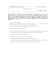Exam 3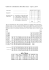10.1\\\-10.3 review ans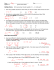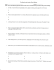homework 4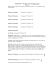Math 0120 Examination #3 Sample Name (Print)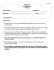Math 30G – Calculus – Sample Final Exam Fall 2012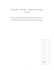Assignment 2 the 1.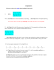Blank PDF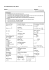3w 3 t5+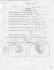Sample Exam 3 - People Pages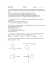2 for 1 Dinner Menu - Club Regent Casino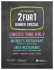Name _____Mr. Perfect________________________________ Date __F 14_______ n l of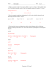Cover Sheet! EXAM2 PRINT your name legibly on the line below.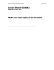Questions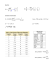Exam 3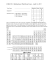Elementary Algebra Sample Test #1 1. 2.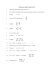AP Chemistry Summer Work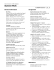The Outsiders: - Mr. Sturgeon`s Wonderful World of English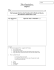CSE 123: Computer Networks Fall Quarter, 2014 MIDTERM EXAM Name